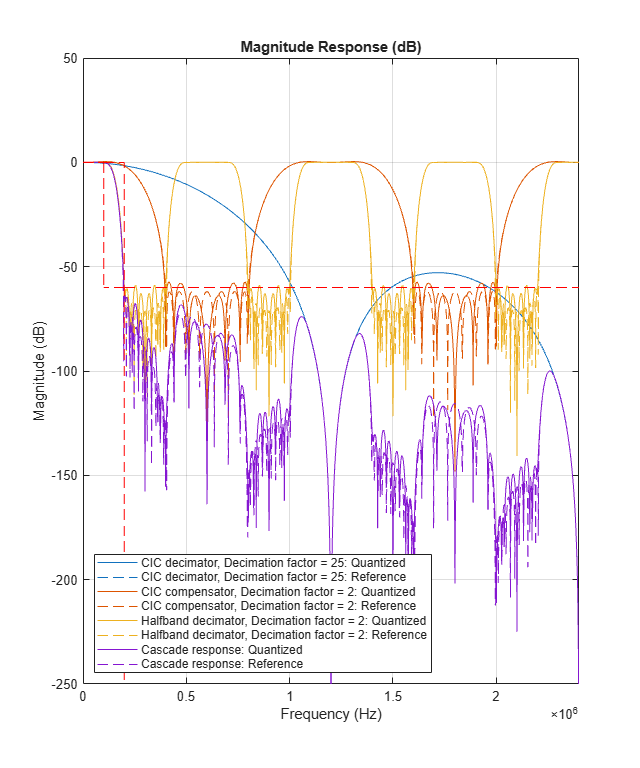# fvtool

Visualize frequency response of digital down converter or digital up converter filter cascade

## Syntax

``fvtool(Conv)``
``fvtool(Conv,'Arithmetic',arithType)``

## Description

````fvtool(Conv)` plots the magnitude response of a digital down converter or digital up converter, `Conv`. By default, the object plots the cascade response up to the second CIC null frequency (or to the first when only one CIC null exists). To use this syntax, the object `Conv` must be locked.```

example

````fvtool(Conv,'Arithmetic',arithType)` specifies the arithmetic type of the filters inside the converter. Set the `'Arithmetic'` input to `'double'`, `'single'`, or `'fixed-point'`. When the `Conv` object is in an unlocked state, you must specify the arithmetic type. When the `Conv` object is in a locked state, it ignores the arithmetic input argument.For example, to plot the magnitude response of a digital down converter in an unlocked state, set the `'Arithmetic'` input. dwnConv = dsp.DigitalDownConverter fvtool(dwnConv,'Arithmetic','fixed-point')```

## Examples

collapse all

Plot the magnitude response of the digital down converter using the `fvtool` function and the `visualizeFilterStages` function.

Create a `dsp.DigitalDownConverter` System object with the default settings. Using the `fvtool `function, plot the magnitude response of the overall filter cascade. The `visualizeFilterStages` function in addition plots the magnitude response of the individual filters stages.

`dwnConv = dsp.DigitalDownConverter`
```dwnConv = dsp.DigitalDownConverter with properties: DecimationFactor: 100 MinimumOrderDesign: true Bandwidth: 200000 StopbandFrequencySource: 'Auto' PassbandRipple: 0.1000 StopbandAttenuation: 60 Oscillator: 'Sine wave' CenterFrequency: 14000000 SampleRate: 30000000 Show all properties ```

Using `fvtool`

If the System object is unlocked, you must specify the filter arithmetic through the `'Arithmetic'` input of the `fvtool` function. If the System object is locked, the arithmetic input is ignored.

`fvtool(dwnConv,'Arithmetic','fixed-point')`Using `visualizeFilterStages`

To view the magnitude response of the individual filter stages, call the `visualizeFilterStages` function.

`visualizeFilterStages(dwnConv,'Arithmetic','fixed-point')`## Input Arguments

collapse all

Digital down converter or digital up converter, specified as a `dsp.DigitalDownConverter` or `dsp.DigitalUpConverter` System object™.

When the `Conv` object is in an unlocked state, you must specify the arithmetic type. When the `Conv` object is in a locked state, it ignores the arithmetic input argument.

Watch now